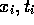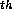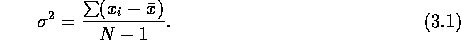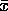Next: Periodogram Up: Period Determination Previous: Period Determination

## Phase Dispersion Minimization

In the PDM method, the period producing the least possible scatter about the derived light curve is chosen. One does this by minimizing the sum of the squares of the differences in the ordinate from one data point to the next. The period resulting in the smallest sum is taken to be the true periods. Conceptually, this has been referred to as the ``shortest string'' connecting all the data points (Dworetsky 1983). More rigorously, any set of observations can be represented by the ordered pair () whererepresent the magnitude and time. of the iobservation. Given N observations, the variance of x is defined as:For this case,is the mean magnitude. One can calculate such a variance for any given sample range. One is interested in minimizing the variance of the data with respect to the mean value of the lightcurve. To do this, one chooses a test period. Modular division of the observation times by this test period assigns a phase to each data point. The observations are then grouped into bins of roughly the same phase. The variance in each bin can now be calculated. The overall variance is the sum of the variance of the samples: The data are explicitly folded of each test period until the total variance is minimized. PDM is well suited to small and randomly spaced samples. This method has no preference for a particular shape (e.g., sinusoidal) for the curve.

Scott J. Wolk
Thu Dec 5 11:30:29 EST 1996